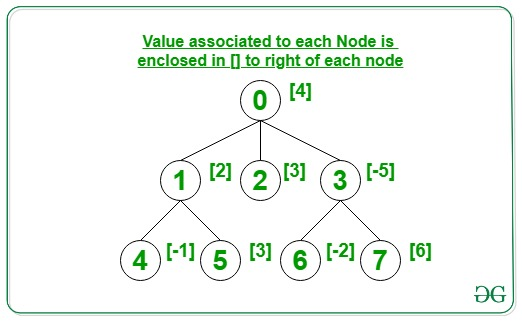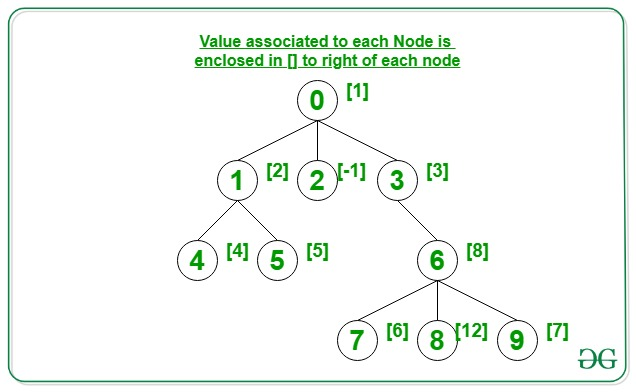Related Articles
Maximum value at each level in an N-ary Tree
• Last Updated : 03 Nov, 2020

Given a N-ary Tree consisting of nodes valued in the range [0, N – 1] and an array arr[] where each node i is associated to value arr[i], the task is to print the maximum value associated with any node at each level of the given N-ary Tree.

Examples:

Input: N = 8, Edges[][] = {{0, 1}, {0, 2}, {0, 3}, {1, 4}, {1, 5}, {3, 6}, {6, 7}},
arr[] = {4, 2, 3, -5, -1, 3, -2, 6}
Output: 4 3 6
Explanation:
Below is the given N-ary Tree:The Max of all nodes of the 0th level is 4.
The Max of all nodes of the 1st level is 3.
The Max of all nodes of the 2nd level is 6.

Input: N = 10, Edges[][] = {{0, 1}, {0, 2}, {0, 3}, {1, 4}, {1, 5}, {3, 6}, {6, 7}, {6, 8}, {6, 9}},
arr[] = {1, 2, -1, 3, 4, 5, 8, 6, 12, 7}
Output: 1 3 8 12
Explanation:
Below is the given N-ary Tree:The Max of all nodes of the 0th level is 1.
The Max of all nodes of the 1st level is 3.
The Max of all nodes of the 2nd level is 8.
The Max of all nodes of the 3rd level is 12.

Approach: This problem can be solved by performing the Level Order Traversal of the given tree. While traversing the tree, process nodes of each level separately. For every level being processed, compute the maximum value of all nodes in the level. Follow the steps below:

1. Store all the child nodes of the current level in a Queue and pop the nodes of the current level one by one.
2. Find the maximum value of all the popped nodes of the current level.
3. Print the maximum value obtained in the above step.
4. Follow the above steps for each level of the given Tree and print the maximum value for each level respectively.

Below is the implementation of the above approach:

## C++

 `// C++ program for the above approach`   `#include ` `using` `namespace` `std;`   `// Function to find the maximum value` `// at each level of N-ary tree` `int` `maxAtLevel(``int` `N, ``int` `M,` `               ``vector<``int``> Value,` `               ``int` `Edges[])` `{` `    ``// Stores the adjacency list` `    ``vector<``int``> adj[N];`   `    ``// Create the adjacency list` `    ``for` `(``int` `i = 0; i < M; i++) {` `        ``int` `u = Edges[i];` `        ``int` `v = Edges[i];` `        ``adj[u].push_back(v);` `    ``}`   `    ``// Perform level order traversal` `    ``// of nodes at each level` `    ``queue<``int``> q;`   `    ``// Push the root node` `    ``q.push(0);`   `    ``// Iterate until queue is empty` `    ``while` `(!q.empty()) {`   `        ``// Get the size of queue` `        ``int` `count = q.size();`   `        ``int` `maxVal = 0;`   `        ``// Iterate for all the nodes` `        ``// in the queue currently` `        ``while` `(count--) {`   `            ``// Dequeue an node from queue` `            ``int` `temp = q.front();` `            ``q.pop();`   `            ``maxVal = max(maxVal,` `                         ``Value[temp]);`   `            ``// Enqueue the children of` `            ``// dequeued node` `            ``for` `(``int` `i = 0;` `                 ``i < adj[temp].size();` `                 ``i++) {` `                ``q.push(adj[temp][i]);` `            ``}` `        ``}`   `        ``// Print the result` `        ``cout << maxVal << ``" "``;` `    ``}` `}`   `// Driver Code` `int` `main()` `{` `    ``// Number of nodes` `    ``int` `N = 10;`   `    ``// Edges of the N-ary tree` `    ``int` `Edges[] = { { 0, 1 }, { 0, 2 },` `                       ``{ 0, 3 }, { 1, 4 },` `                       ``{ 1, 5 }, { 3, 6 },` `                       ``{ 6, 7 }, { 6, 8 },` `                       ``{ 6, 9 } };`   `    ``// Given cost` `    ``vector<``int``> Value = { 1, 2, -1, 3, 4,` `                          ``5, 8, 6, 12, 7 };`   `    ``// Function Call` `    ``maxAtLevel(N, N - 1, Value, Edges);`   `    ``return` `0;` `}`

## Java

 `// Java program for ` `// the above approach` `import` `java.util.*;` `class` `GFG{`   `// Function to find the maximum value` `// at each level of N-ary tree` `static` `void` `maxAtLevel(``int` `N, ``int` `M,` `                       ``int` `[]Value,` `                       ``int` `Edges[][])` `{` `  ``// Stores the adjacency list` `  ``Vector []adj = ``new` `Vector[N];` `  `  `  ``for` `(``int` `i = ``0``; i < adj.length; i++)` `    ``adj[i] = ``new` `Vector();`   `  ``// Create the adjacency list` `  ``for` `(``int` `i = ``0``; i < M; i++) ` `  ``{` `    ``int` `u = Edges[i][``0``];` `    ``int` `v = Edges[i][``1``];` `    ``adj[u].add(v);` `  ``}`   `  ``// Perform level order traversal` `  ``// of nodes at each level` `  ``Queue q = ``new` `LinkedList<>();`   `  ``// Push the root node` `  ``q.add(``0``);`   `  ``// Iterate until queue is empty` `  ``while` `(!q.isEmpty()) ` `  ``{` `    ``// Get the size of queue` `    ``int` `count = q.size();`   `    ``int` `maxVal = ``0``;`   `    ``// Iterate for all the nodes` `    ``// in the queue currently` `    ``while` `(count-- > ``0``) ` `    ``{` `      ``// Dequeue an node from queue` `      ``int` `temp = q.peek();` `      ``q.remove();`   `      ``maxVal = Math.max(maxVal, Value[temp]);`   `      ``// Enqueue the children of` `      ``// dequeued node` `      ``for` `(``int` `i = ``0``;` `               ``i < adj[temp].size(); i++) ` `      ``{` `        ``q.add(adj[temp].get(i));` `      ``}` `    ``}`   `    ``// Print the result` `    ``System.out.print(maxVal + ``" "``);` `  ``}` `}`   `// Driver Code` `public` `static` `void` `main(String[] args)` `{` `  ``// Number of nodes` `  ``int` `N = ``10``;`   `  ``// Edges of the N-ary tree` `  ``int` `Edges[][] = {{``0``, ``1``}, {``0``, ``2``},` `                   ``{``0``, ``3``}, {``1``, ``4``},` `                   ``{``1``, ``5``}, {``3``, ``6``},` `                   ``{``6``, ``7``}, {``6``, ``8``},` `                   ``{``6``, ``9``}};`   `  ``// Given cost` `  ``int` `[]Value = {``1``, ``2``, -``1``, ``3``, ``4``,` `                 ``5``, ``8``, ``6``, ``12``, ``7``};`   `  ``// Function Call` `  ``maxAtLevel(N, N - ``1``, Value, Edges);` `}` `}`   `// This code is contributed by 29AjayKumar`

## Python3

 `# Python3 program for the above approach`   `# Function to find the maximum value` `# at each level of N-ary tree` `def` `maxAtLevel(N, M, Value, Edges):` `    `  `    ``# Stores the adjacency list` `    ``adj ``=` `[[] ``for` `i ``in` `range``(N)]`   `    ``# Create the adjacency list` `    ``for` `i ``in` `range``(M):` `        ``u ``=` `Edges[i][``0``]` `        ``v ``=` `Edges[i][``1``]` `        ``adj[u].append(v)`   `    ``# Perform level order traversal` `    ``# of nodes at each level` `    ``q ``=` `[]`   `    ``# Push the root node` `    ``q.append(``0``)`   `    ``# Iterate until queue is empty` `    ``while` `(``len``(q)):` `        `  `        ``# Get the size of queue` `        ``count ``=` `len``(q)`   `        ``maxVal ``=` `0`   `        ``# Iterate for: all the nodes` `        ``# in the queue currently` `        ``while` `(count):` `            `  `            ``# Dequeue an node from queue` `            ``temp ``=` `q[``0``]` `            ``q.remove(q[``0``])`   `            ``maxVal ``=` `max``(maxVal, Value[temp])`   `            ``# Enqueue the children of` `            ``# dequeued node` `            ``for` `i ``in` `range``(``len``(adj[temp])):` `                ``q.append(adj[temp][i])` `                `  `            ``count ``-``=` `1`   `        ``# Print the result` `        ``print``(maxVal, end ``=` `" "``)`   `# Driver Code` `if` `__name__ ``=``=` `'__main__'``:` `    `  `    ``# Number of nodes` `    ``N ``=` `10`   `    ``# Edges of the N-ary tree` `    ``Edges ``=` `[ [ ``0``, ``1` `], [ ``0``, ``2` `],` `              ``[ ``0``, ``3` `], [ ``1``, ``4` `],` `              ``[ ``1``, ``5` `], [ ``3``, ``6` `],` `              ``[ ``6``, ``7` `], [ ``6``, ``8` `],` `              ``[ ``6``, ``9` `] ]`   `    ``# Given cost` `    ``Value ``=` `[ ``1``, ``2``, ``-``1``, ``3``, ``4``, ` `              ``5``, ``8``, ``6``, ``12``, ``7` `]`   `    ``# Function Call` `    ``maxAtLevel(N, N ``-` `1``, Value, Edges)`   `# This code is contributed by ipg2016107`

## C#

 `// C# program for ` `// the above approach` `using` `System;` `using` `System.Collections.Generic;` `class` `GFG{`   `// Function to find the ` `// maximum value at each ` `// level of N-ary tree` `static` `void` `maxAtLevel(``int` `N, ``int` `M,` `                       ``int` `[]Value,` `                       ``int` `[,]Edges)` `{` `  ``// Stores the adjacency list` `  ``List<``int``> []adj = ``new` `List<``int``>[N];`   `  ``for` `(``int` `i = 0; i < adj.Length; i++)` `    ``adj[i] = ``new` `List<``int``>();`   `  ``// Create the adjacency list` `  ``for` `(``int` `i = 0; i < M; i++) ` `  ``{` `    ``int` `u = Edges[i, 0];` `    ``int` `v = Edges[i, 1];` `    ``adj[u].Add(v);` `  ``}`   `  ``// Perform level order traversal` `  ``// of nodes at each level` `  ``Queue<``int``> q = ``new` `Queue<``int``>();`   `  ``// Push the root node` `  ``q.Enqueue(0);`   `  ``// Iterate until queue is empty` `  ``while` `(q.Count != 0) ` `  ``{` `    ``// Get the size of queue` `    ``int` `count = q.Count;`   `    ``int` `maxVal = 0;`   `    ``// Iterate for all the nodes` `    ``// in the queue currently` `    ``while` `(count-- > 0) ` `    ``{` `      ``// Dequeue an node from queue` `      ``int` `temp = q.Peek();` `      ``q.Dequeue();`   `      ``maxVal = Math.Max(maxVal, ` `                        ``Value[temp]);`   `      ``// Enqueue the children of` `      ``// dequeued node` `      ``for` `(``int` `i = 0;` `               ``i < adj[temp].Count; i++) ` `      ``{` `        ``q.Enqueue(adj[temp][i]);` `      ``}` `    ``}`   `    ``// Print the result` `    ``Console.Write(maxVal + ``" "``);` `  ``}` `}`   `// Driver Code` `public` `static` `void` `Main(String[] args)` `{` `  ``// Number of nodes` `  ``int` `N = 10;`   `  ``// Edges of the N-ary tree` `  ``int` `[,]Edges = {{0, 1}, {0, 2},` `                  ``{0, 3}, {1, 4},` `                  ``{1, 5}, {3, 6},` `                  ``{6, 7}, {6, 8},` `                  ``{6, 9}};`   `  ``// Given cost` `  ``int` `[]Value = {1, 2, -1, 3, 4,` `                 ``5, 8, 6, 12, 7};`   `  ``// Function Call` `  ``maxAtLevel(N, N - 1, Value, Edges);` `}` `}`   `// This code is contributed by 29AjayKumar`

Output:

```1 3 8 12

```

Time Complexity: O(N)
Auxiliary Space: O(N)

Attention reader! Don’t stop learning now. Get hold of all the important DSA concepts with the DSA Self Paced Course at a student-friendly price and become industry ready.

My Personal Notes arrow_drop_up
Recommended Articles
Page :